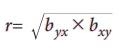Home | | Business Maths 11th std | Summary of Correlation and Regression analysis

# Summary of Correlation and Regression analysis

The term correlation refers to the degree of relationship between two or more variables.

SUMMARY

The term correlation refers to the degree of relationship between two or more variables.

Scatter diagram is a graphic device for finding correlation between two variables.

Karl Pearson correlation coefficient r(x,y)=Correlation coefficient r lies between ŌĆō1 and 1. (i.e) ŌĆō1 =< r =< 1

When r=+1 , then the correlation is perfect positive

When r=ŌĆō1 , then the correlation is perfect negative

When r=0, then there is no relationship between the variables, (i.e) the variables are uncorrelated.

Rank correlation deals with qualitative characteristics.

SpearmanŌĆÖs rank correlation coefficient formula Žü is given bywhere d = The difference between two ranks = RXŌĆōRY

N = Number of paired observations.

Correlation represents linear relationship between the variables but the regression helps to estimate (or predict) one variable by using the other variable.

Regression lines ofThe two regression lines passing through their respective means of X and Y

Calculation of the regression coefficients.The Properties of regression coefficients.

(i)(ii) both the regression coefficients cannot be greater than one.

(iii) Both the regression coefficients have same sign.

Tags : Maths , 11th Business Mathematics and Statistics(EMS) : Chapter 9 : Correlation and Regression analysis
Study Material, Lecturing Notes, Assignment, Reference, Wiki description explanation, brief detail
11th Business Mathematics and Statistics(EMS) : Chapter 9 : Correlation and Regression analysis : Summary of Correlation and Regression analysis | Maths